## Saturday, 9 August 2014

### chapter 14 Worked Out Examples 5

 Example: 7
Evaluate the following limits:
 (a)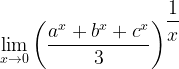$\mathop {\lim }\limits_{x \to 0} {\left( {\dfrac{{{a^x} + {b^x} + {c^x}}}{3}} \right)^{\dfrac{1}{x}}}$ (b)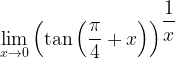$\mathop {\lim }\limits_{x \to 0} {\left( {\tan \left( {\dfrac{\pi }{4} + x} \right)} \right)^{\dfrac{1}{x}}}$ (c)$\mathop {\lim }\limits_{x \to 0} {\left( {\dfrac{{\sin x}}{x}} \right)^{\dfrac{1}{{{x^2}}}}}$ (d)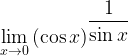$\mathop {\lim }\limits_{x \to 0} {(\cos x)^{\dfrac{1}{{\sin x}}}}$ (e)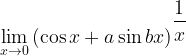$\mathop {\lim }\limits_{x \to 0} {\left( {\cos x + a\sin bx} \right)^{\dfrac{1}{x}}}$
 Solution: 7-(a)
Notice that all the limits above are of the form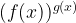${(f(x))^{g(x)}}$ where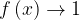$f\left( x \right) \to 1$and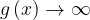$g\left( x \right) \to \infty$ that is, these limits are of the indeterminate form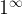${1^\infty }$.
In Section –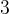$3$, we saw how to evaluate such limits. Writing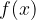$f(x)$ as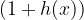$(1 + h(x))$ reduces this limit to , where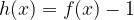$h(x) = f(x) - 1$.
We will now directly apply this result to evaluate the limits above.$\mathop {\lim }\limits_{x \to 0} {\left( {\dfrac{{{a^x} + {b^x} + {c^x}}}{3}} \right)^{\dfrac{1}{x}}}$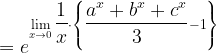$= {e^{\mathop {\lim }\limits_{x \to 0} \dfrac{1}{x} \cdot \left\{ {\dfrac{{{a^x} + {b^x} + {c^x}}}{3} - 1} \right\}}}$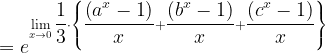$= {e^{\mathop {\lim }\limits_{x \to 0} \dfrac{1}{3} \cdot \left\{ {\dfrac{{\left( {{a^x} - 1} \right)}}{x} + \dfrac{{\left( {{b^x} - 1} \right)}}{x} + \dfrac{{\left( {{c^x} - 1} \right)}}{x}} \right\}}}$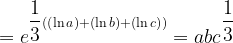$= {e^{\dfrac{1}{3}\left( {\left( {\ln a} \right) + (\ln b) + (\ln c)} \right)}} = ab{c^{\dfrac{1}{3}}}$
 Solution: 7-(b)
\$\mathop {\lim }\limits_{x \to 0} {\left( {\tan \left( {\dfrac{\pi }{4} + x} \right)} \right)^{\dfrac{1}{x}}}$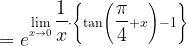$= {e^{\mathop {\lim }\limits_{x \to 0} \dfrac{1}{x} \cdot \left\{ {\tan \left( {\dfrac{\pi }{4} + x} \right) - 1} \right\}}}$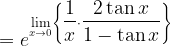$= {e^{\mathop {\lim }\limits_{x \to 0} \left\{ {\dfrac{1}{x} \cdot \dfrac{{2\tan x}}{{1 - \tan x}}} \right\}}}$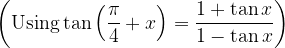$\left( {{\rm{Using}}\tan \left( {\dfrac{\pi }{4} + x} \right) = \dfrac{{1 + \tan x}}{{1 - \tan x}}} \right)$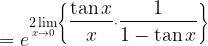$= {e^{\mathop {2\lim }\limits_{x \to 0} \left\{ {\dfrac{{\tan x}}{x} \cdot \dfrac{1}{{1 - \tan x}}} \right\}}}$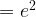$= {e^2}$
 Solution: 7-(c)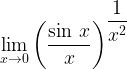$\mathop {\lim }\limits_{x \to 0} {\left( {\dfrac{{\sin \,x}}{x}} \right)^{\dfrac{1}{{{x^2}}}}}$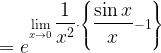$= {e^{\mathop {\lim }\limits_{x \to 0} \dfrac{1}{{{x^2}}} \cdot \left\{ {\dfrac{{\sin x}}{x} - 1} \right\}}}$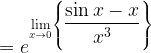$= {e^{\mathop {\lim }\limits_{x \to 0} \left\{ {\dfrac{{\sin x - x}}{{{x^3}}}} \right\}}}$
In example -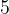$5$ Part –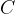$C$, we considered the limit in the exponent above.
The value of this limit is therefore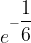${e^{ - \dfrac{1}{6}}}$
 Solution: 7-(d)$\mathop {\lim }\limits_{x \to 0} {(\cos x)^{\dfrac{1}{{\sin x}}}}$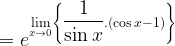$= {e^{\mathop {\lim }\limits_{x \to 0} \left\{ {\dfrac{1}{{\sin x}}.(\cos x - 1)} \right\}}}$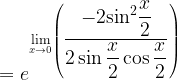$= {e^{\mathop {\lim }\limits_{x \to 0} \left( {\dfrac{{ - 2{{\sin }^2}\dfrac{x}{2}}}{{2\sin \dfrac{x}{2}\cos \dfrac{x}{2}}}} \right)}}$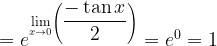$= {e^{\mathop {\lim }\limits_{x \to 0} \left( {\dfrac{{ - \tan x}}{2}} \right)}} = {e^0} = 1$
 Solution: 7-(e)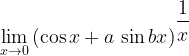$\mathop {\lim }\limits_{x \to 0} {\left( {\cos x + a\,\sin bx} \right)^{\dfrac{1}{x}}}$$= {e^{\mathop {\lim }\limits_{x \to 0} \frac{1}{x} \cdot \left\{ {\cos x + a\,\sin bx - 1} \right\}}}$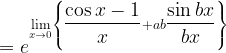$= {e^{\mathop {\lim }\limits_{x \to 0} \left\{ {\dfrac{{\cos x - 1}}{x} + ab\dfrac{{\sin bx}}{{bx}}} \right\}}}$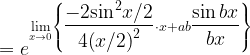$= {e^{\mathop {\lim }\limits_{x \to 0} \left\{ {\dfrac{{ - 2{{\sin }^2}x/2}}{{4{{\left( {x/2} \right)}^2}}} \cdot x + ab\dfrac{{\sin bx}}{{bx}}} \right\}}}$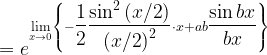$= {e^{\mathop {\lim }\limits_{x \to 0} \left\{ { - \dfrac{1}{2}\dfrac{{{{\sin }^2}\left( {x/2} \right)}}{{{{\left( {x/2} \right)}^2}}} \cdot x + ab\dfrac{{\sin bx}}{{bx}}} \right\}}}$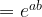$= {e^{ab}}$